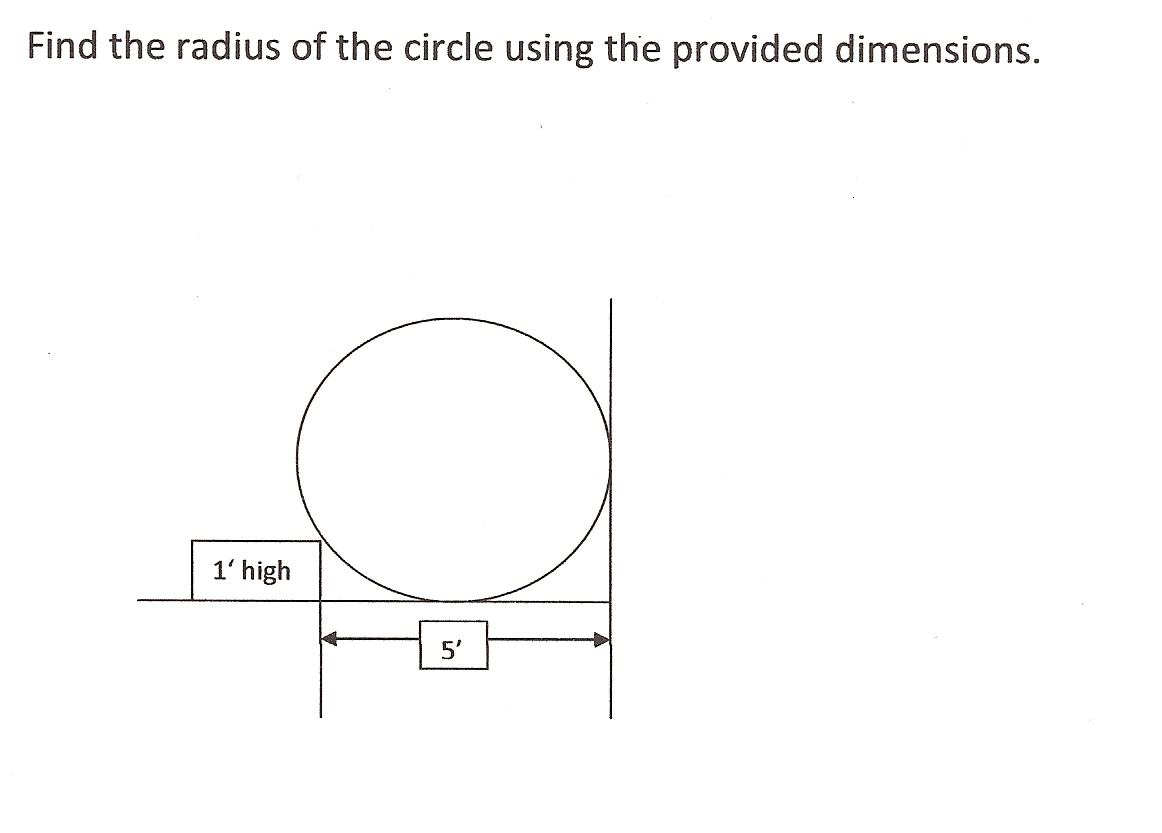# Need to find the radiusAny assistance much appreciated.Note by Monty McGee
3 years, 5 months ago

This discussion board is a place to discuss our Daily Challenges and the math and science related to those challenges. Explanations are more than just a solution — they should explain the steps and thinking strategies that you used to obtain the solution. Comments should further the discussion of math and science.

When posting on Brilliant:

• Use the emojis to react to an explanation, whether you're congratulating a job well done , or just really confused .
• Ask specific questions about the challenge or the steps in somebody's explanation. Well-posed questions can add a lot to the discussion, but posting "I don't understand!" doesn't help anyone.
• Try to contribute something new to the discussion, whether it is an extension, generalization or other idea related to the challenge.

MarkdownAppears as
*italics* or _italics_ italics
**bold** or __bold__ bold
- bulleted- list
• bulleted
• list
1. numbered2. list
1. numbered
2. list
Note: you must add a full line of space before and after lists for them to show up correctly
paragraph 1paragraph 2

paragraph 1

paragraph 2

[example link](https://brilliant.org)example link
> This is a quote
This is a quote
    # I indented these lines
# 4 spaces, and now they show
# up as a code block.

print "hello world"
# I indented these lines
# 4 spaces, and now they show
# up as a code block.

print "hello world"
MathAppears as
Remember to wrap math in $$ ... $$ or $ ... $ to ensure proper formatting.
2 \times 3 $2 \times 3$
2^{34} $2^{34}$
a_{i-1} $a_{i-1}$
\frac{2}{3} $\frac{2}{3}$
\sqrt{2} $\sqrt{2}$
\sum_{i=1}^3 $\sum_{i=1}^3$
\sin \theta $\sin \theta$
\boxed{123} $\boxed{123}$

Sort by:

Have tried in vain to find the radius.

- 3 years, 5 months ago

Hint 1: Try forming a right triangle.

Hint 2: Let $O$ be the center of the circle and $X$ be the point where the rectangle touches the circle. Create a right triangle with $OX$ as the hypotenuse and whose side lengths you can easily find.

- 3 years, 5 months ago

Thanks, Steven. I tried that before but obviously did not hit upon the correct setup; will go at it again.

Monty

- 3 years, 5 months ago

You are assuming that rectangle touches the circle, when the illustration shows that it doesn't.

- 3 years, 5 months ago

That's my fault; I didn't draw it correctly. The rectangle is indeed supposed to be touching the circle.

- 3 years, 5 months ago

I don't think there is enough info to solve the problem. That's not a circle, it's an oval. It's wider than it is tall. It won't have 1 radius.

- 3 years, 5 months ago

The drawing is not correct; I didn't draw it correctly with the Word drawing tool; it is supposed to be a circle.

- 3 years, 5 months ago

sqrt [ r^2 - (r-1)^2 ] + r = 5;

r = 6 +/- sqrt(10);

r = 6 - sqrt(10);

r = 2.84

- 3 years, 3 months ago

- 3 years, 5 months ago

Hi Annie. Thanks for the comment. Who is Siri ?

- 3 years, 5 months ago

Oh know, the apple help system. Here is some information about it: Siri

- 3 years, 5 months ago

Thanks.

- 3 years, 5 months ago

No probs. Just remember to thank Siri

- 3 years, 5 months ago